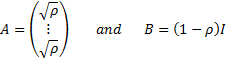/

### Showing that a Gaussian copula is not in general an Archimdean copula

An-dimensional Archimedean copula is one that can be represented by: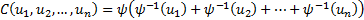One way of showing that the Gaussian copula is not in general an Archimedean copula is to consider a three dimensional Gaussian copula. Its copula density (for a correlation matrix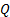) can be written as: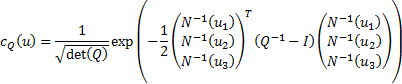In general,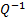will have 3 different off-diagonal elements, derived from the three different correlations between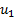and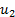, betweenand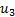and betweenandrespectively. Thus the form of the copula density if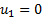expressed as a function of the remaining two components of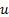, i.e. hereand, will differ from its form if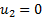expressed as a function ofandetc. However, to be Archimedean, the copula needs to be indifferent between the components of.

For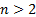, the Gaussian copula has too many free parameters to be Archimedean.

Conversely, if returns are multivariate normal and have an exchangeable copula then the returns can be characterised by a factor structure involving a single factor.

A set of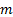random variables,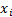(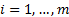) is said to possess a factor structure if their covariance matrix,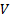, is of the form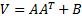whereis an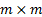matrix,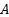is an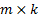matrix (and there are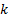factors) and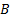is a diagonal matrix. Suppose the variance of eachis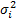and we define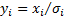. Then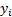have unit variance and their covariance (now also correlation) matrix also has the form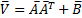. The copulas describing theandare the same. If it is exchangeable andare multivariate normal then we must have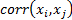being the same for all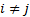, say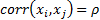. This arises if we setandas follows, ifis the identity matrix: